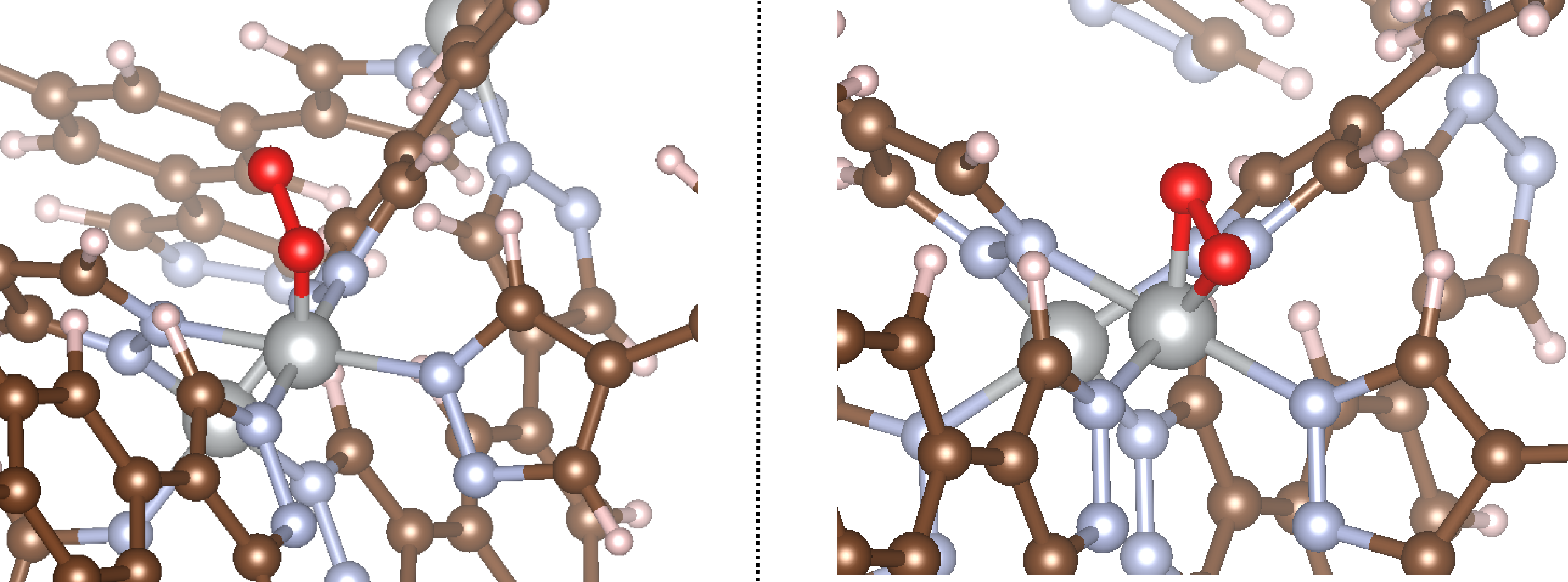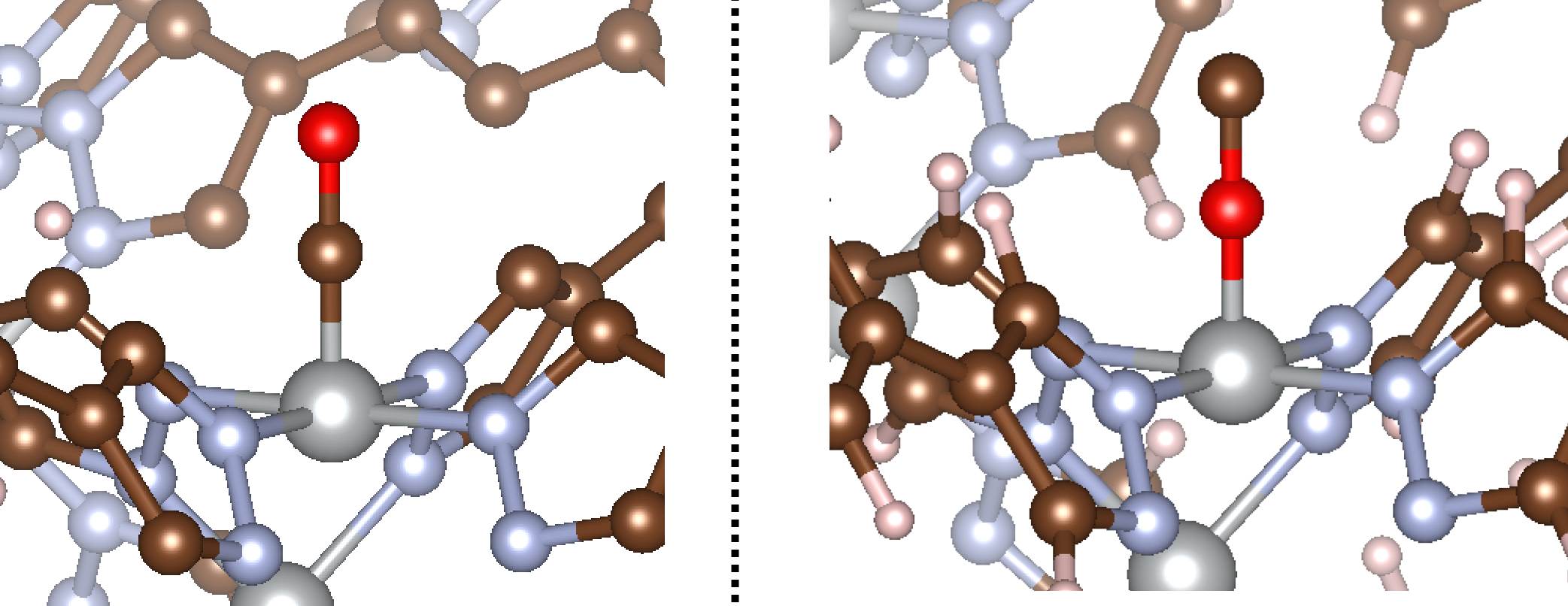# Diatomics¶

In this example, we’ll work through how to add two-atom adsorbates to open metal sites in MOFs. The CIF for the MOF we’ll use for this example can be found `here`. This MOF is known Ni3(BTP)2, or Ni-BTP for short, and has a sodalite-like structure with square planar Ni cations.

## Homoatomic¶

For this example, we will consider the initialization of an O2 molecule to a single coordinatively unsaturated Ni site. O2 can bind in an end-on (η1-O) or side-on (η2-O) mode depending on the structure. We’ll consider both for this example. The code to handle this is shown below.

```import os

mof_path = os.path.join('example_MOFs','Ni-BTP.cif') #path to CIF of MOF

d_X1X2=1.2,ang_MX1X2=120)

d_X1X2=1.2,ang_MX1X2=90)
```

Like with the monatomic example, we need to initialize an `adsorbate_constructor` object and then provide it the MOF of interest. In the case of diatomics, we have a few new keywords to introduce. In addition to the arguments described in the monatomic tutorial, we now need to be able to tell MAI what kind of denticity we would like (i.e. end-on or side-on adsorption) and what we want the X1-X2 bond length and M-X1-X2 bond angle to be (if X1-X2 is our diatomic of interest and M is our metal adsorption site). The arguments used here are described below:

1. The `ads` argument is a string of the molecule that you want to add to the structure. Note that MAI will internally strip any characters following (and including) an underscore, so `ads='O2_end'` and `ads='O2_side'` both get stripped to ‘O2’. That being said, the full string for the `ads` argument will be used when writing the filenames of the new CIFs, so using an underscore can be helpful for organizational purposes.
2. The `d_MX1` argument is the desired distance between the adsorption site (i.e. the Ni species) and the adsorbate (in Å). If the adsorbate is bound in an end-on fashion, this represents the M-X1 distance. If the adsorbate is bound in a side-on fashion, this represents the distance between M and the midpoint between X1 and X2. Here, we set `d_MX1=1.5`.
3. The `eta` keyword argument is an integer representing the denticity. In other words, `eta=1` would be an end-on adsorption mode, whereas `eta=2` would be a side-on adsorption mode. By default, `eta=1` if unspecified. For this example, we decided to explore both options.
4. The `d_X1X2` keyword argument is the desired distance between X1 and X2 (in Å). If not specified, it will default to the value for `d_MX1`. Here, we decided to set `d_X1X2=1.2`, which is a reasonable O-O bond distance.
5. The `ang_MX1X2` keyword argument is the angle between the adsorption site and the adsorbate (in degrees). If the adsorbate is bound in an end-on fashion, this represents the M-X1-X2 bond angle. If the adsorbate is bound in a side-on fashion, this represents the angle between M, the midpoint between X1 and X2, and X2. By default, it assumes `ang_MX1X2=180` if `eta=1` and `ang_MX1X2=90` if `eta=2`. For this example, we use `ang_MX1X2=120` and `ang_MX1X2=90`, respectively, which is representative of common O2 binding geometries.

That takes care of initializing the `adsorbate_constructor` object. With this, we provide the object with the path to the MOF and the site index, and it will initialize the adsorbate for us. Now let’s see what happens as a result of running this code! The initialized structures are shown below:Exactly what we’d expect yet again! You can see that in the first example, O2 is bound end-on, whereas in the second it is bound side-on, as specified in the example script. The bond angles and distances are the same as those specified in the input file.

## Heteroatomic¶

MAI also supports heteratomic adsorbates. In this example, we’ll consider the adsorption of a single CO molecule with the same MOF. The only thing that changes for heteroatomic adsorbates is that you need to tell MAI which atom is the “connecting atom” (i.e. the atom of the adsorbate bound to the metal adsorption site) if bound in an end-on fashion. By default, MAI will assume that the first atom in `ads` is the connecting atom. Therefore, setting `ads='CO'` or `ads='OC'` would yield M-C-O or M-O-C binding modes, respectively.

```import os

mof_path = os.path.join('example_MOFs','Ni-BTP.cif') #path to CIF of MOF